Work, Energy, and Energy Resources

# Conservation of Energy

OpenStaxCollege

### Learning Objectives

• Explain the law of the conservation of energy.
• Describe some of the many forms of energy.
• Define efficiency of an energy conversion process as the fraction left as useful energy or work, rather than being transformed, for example, into thermal energy.

# Law of Conservation of Energy

Energy, as we have noted, is conserved, making it one of the most important physical quantities in nature. The law of conservation of energy can be stated as follows:

Total energy is constant in any process. It may change in form or be transferred from one system to another, but the total remains the same.

We have explored some forms of energy and some ways it can be transferred from one system to another. This exploration led to the definition of two major types of energy—mechanical energy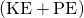and energy transferred via work done by nonconservative forces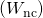. But energy takes many other forms, manifesting itself in many different ways, and we need to be able to deal with all of these before we can write an equation for the above general statement of the conservation of energy.

# Other Forms of Energy than Mechanical Energy

At this point, we deal with all other forms of energy by lumping them into a single group called other energy (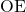). Then we can state the conservation of energy in equation form as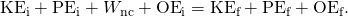All types of energy and work can be included in this very general statement of conservation of energy. Kinetic energy is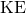, work done by a conservative force is represented by, work done by nonconservative forces is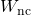, and all other energies are included as. This equation applies to all previous examples; in those situationswas constant, and so it subtracted out and was not directly considered.

Making Connections: Usefulness of the Energy Conservation Principle

The fact that energy is conserved and has many forms makes it very important. You will find that energy is discussed in many contexts, because it is involved in all processes. It will also become apparent that many situations are best understood in terms of energy and that problems are often most easily conceptualized and solved by considering energy.

When doesplay a role? One example occurs when a person eats. Food is oxidized with the release of carbon dioxide, water, and energy. Some of this chemical energy is converted to kinetic energy when the person moves, to potential energy when the person changes altitude, and to thermal energy (another form of).

# Some of the Many Forms of Energy

What are some other forms of energy? You can probably name a number of forms of energy not yet discussed. Many of these will be covered in later chapters, but let us detail a few here. Electrical energy is a common form that is converted to many other forms and does work in a wide range of practical situations. Fuels, such as gasoline and food, carry chemical energy that can be transferred to a system through oxidation. Chemical fuel can also produce electrical energy, such as in batteries. Batteries can in turn produce light, which is a very pure form of energy. Most energy sources on Earth are in fact stored energy from the energy we receive from the Sun. We sometimes refer to this as radiant energy, or electromagnetic radiation, which includes visible light, infrared, and ultraviolet radiation. Nuclear energy comes from processes that convert measurable amounts of mass into energy. Nuclear energy is transformed into the energy of sunlight, into electrical energy in power plants, and into the energy of the heat transfer and blast in weapons. Atoms and molecules inside all objects are in random motion. This internal mechanical energy from the random motions is called thermal energy, because it is related to the temperature of the object. These and all other forms of energy can be converted into one another and can do work.

[link] gives the amount of energy stored, used, or released from various objects and in various phenomena. The range of energies and the variety of types and situations is impressive.

Problem-Solving Strategies for Energy

You will find the following problem-solving strategies useful whenever you deal with energy. The strategies help in organizing and reinforcing energy concepts. In fact, they are used in the examples presented in this chapter. The familiar general problem-solving strategies presented earlier—involving identifying physical principles, knowns, and unknowns, checking units, and so on—continue to be relevant here.

Step 1. Determine the system of interest and identify what information is given and what quantity is to be calculated. A sketch will help.

Step 2. Examine all the forces involved and determine whether you know or are given the potential energy from the work done by the forces. Then use step 3 or step 4.

Step 3. If you know the potential energies for the forces that enter into the problem, then forces are all conservative, and you can apply conservation of mechanical energy simply in terms of potential and kinetic energy. The equation expressing conservation of energy is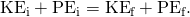Step 4. If you know the potential energy for only some of the forces, possibly because some of them are nonconservative and do not have a potential energy, or if there are other energies that are not easily treated in terms of force and work, then the conservation of energy law in its most general form must be used.In most problems, one or more of the terms is zero, simplifying its solution. Do not calculate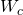, the work done by conservative forces; it is already incorporated in theterms.

Step 5. You have already identified the types of work and energy involved (in step 2). Before solving for the unknown, eliminate terms wherever possible to simplify the algebra. For example, choose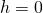at either the initial or final point, so that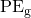is zero there. Then solve for the unknown in the customary manner.

Step 6.Check the answer to see if it is reasonable. Once you have solved a problem, reexamine the forms of work and energy to see if you have set up the conservation of energy equation correctly. For example, work done against friction should be negative, potential energy at the bottom of a hill should be less than that at the top, and so on. Also check to see that the numerical value obtained is reasonable. For example, the final speed of a skateboarder who coasts down a 3-m-high ramp could reasonably be 20 km/h, but not 80 km/h.

# Transformation of Energy

The transformation of energy from one form into others is happening all the time. The chemical energy in food is converted into thermal energy through metabolism; light energy is converted into chemical energy through photosynthesis. In a larger example, the chemical energy contained in coal is converted into thermal energy as it burns to turn water into steam in a boiler. This thermal energy in the steam in turn is converted to mechanical energy as it spins a turbine, which is connected to a generator to produce electrical energy. (In all of these examples, not all of the initial energy is converted into the forms mentioned. This important point is discussed later in this section.)

Another example of energy conversion occurs in a solar cell. Sunlight impinging on a solar cell (see [link]) produces electricity, which in turn can be used to run an electric motor. Energy is converted from the primary source of solar energy into electrical energy and then into mechanical energy.

Solar energy is converted into electrical energy by solar cells, which is used to run a motor in this solar-power aircraft. (credit: NASA)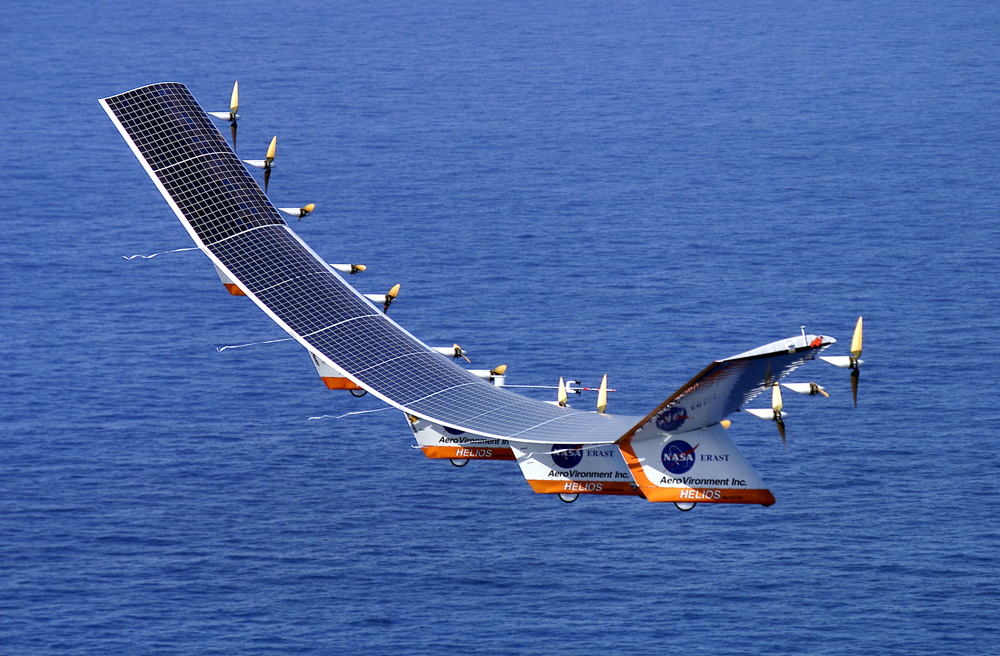Energy of Various Objects and Phenomena
Object/phenomenon Energy in joules
Big Bang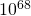Energy released in a supernova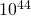Fusion of all the hydrogen in Earth’s oceans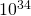Annual world energy use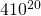Large fusion bomb (9 megaton)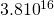1 kg hydrogen (fusion to helium)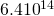1 kg uranium (nuclear fission)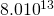Hiroshima-size fission bomb (10 kiloton)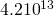90,000-ton aircraft carrier at 30 knots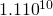1 barrel crude oil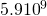1 ton TNT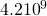1 gallon of gasoline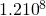Daily home electricity use (developed countries)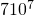Daily adult food intake (recommended)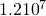1000-kg car at 90 km/h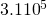1 g fat (9.3 kcal)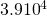ATP hydrolysis reaction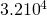1 g carbohydrate (4.1 kcal)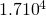1 g protein (4.1 kcal)Tennis ball at 100 km/h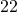Mosquito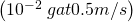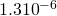Single electron in a TV tube beam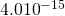Energy to break one DNA strand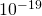# Efficiency

Even though energy is conserved in an energy conversion process, the output of useful energy or work will be less than the energy input. The efficiency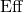of an energy conversion process is defined as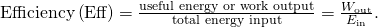[link] lists some efficiencies of mechanical devices and human activities. In a coal-fired power plant, for example, about 40% of the chemical energy in the coal becomes useful electrical energy. The other 60% transforms into other (perhaps less useful) energy forms, such as thermal energy, which is then released to the environment through combustion gases and cooling towers.

Efficiency of the Human Body and Mechanical Devices
Activity/device Efficiency (%)1
Cycling and climbing 20
Swimming, surface 2
Swimming, submerged 4
Shoveling 3
Weightlifting 9
Steam engine 17
Gasoline engine 30
Diesel engine 35
Nuclear power plant 35
Coal power plant 42
Electric motor 98
Compact fluorescent light 20
Gas heater (residential) 90
Solar cell 10
PhET Explorations: Masses and Springs

A realistic mass and spring laboratory. Hang masses from springs and adjust the spring stiffness and damping. You can even slow time. Transport the lab to different planets. A chart shows the kinetic, potential, and thermal energies for each spring.

# Section Summary

• The law of conservation of energy states that the total energy is constant in any process. Energy may change in form or be transferred from one system to another, but the total remains the same.
• When all forms of energy are considered, conservation of energy is written in equation form as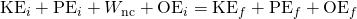, whereis all other forms of energy besides mechanical energy.
• Commonly encountered forms of energy include electric energy, chemical energy, radiant energy, nuclear energy, and thermal energy.
• Energy is often utilized to do work, but it is not possible to convert all the energy of a system to work.
• The efficiencyof a machine or human is defined to be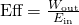, where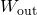is useful work output and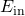is the energy consumed.

# Conceptual Questions

Consider the following scenario. A car for which friction is not negligible accelerates from rest down a hill, running out of gasoline after a short distance. The driver lets the car coast farther down the hill, then up and over a small crest. He then coasts down that hill into a gas station, where he brakes to a stop and fills the tank with gasoline. Identify the forms of energy the car has, and how they are changed and transferred in this series of events. (See [link].)

A car experiencing non-negligible friction coasts down a hill, over a small crest, then downhill again, and comes to a stop at a gas station.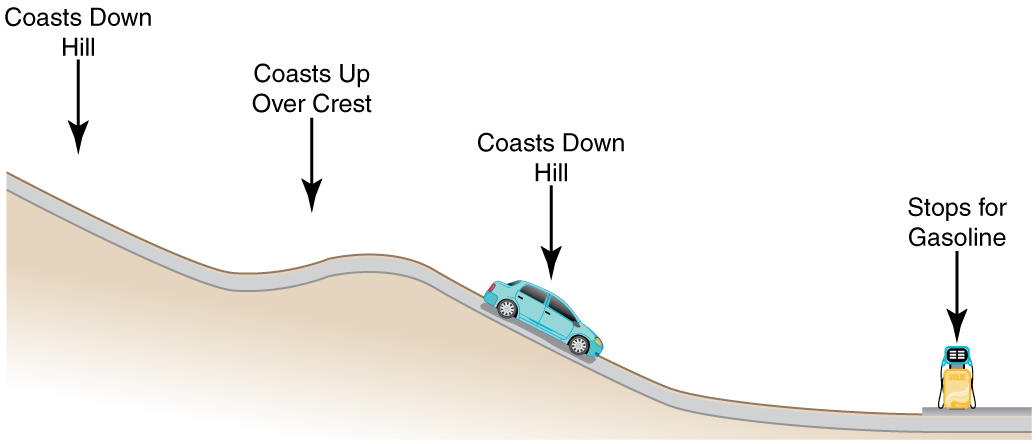Describe the energy transfers and transformations for a javelin, starting from the point at which an athlete picks up the javelin and ending when the javelin is stuck into the ground after being thrown.

Do devices with efficiencies of less than one violate the law of conservation of energy? Explain.

List four different forms or types of energy. Give one example of a conversion from each of these forms to another form.

List the energy conversions that occur when riding a bicycle.

# Problems & Exercises

Using values from [link], how many DNA molecules could be broken by the energy carried by a single electron in the beam of an old-fashioned TV tube? (These electrons were not dangerous in themselves, but they did create dangerous x rays. Later model tube TVs had shielding that absorbed x rays before they escaped and exposed viewers.)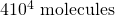Using energy considerations and assuming negligible air resistance, show that a rock thrown from a bridge 20.0 m above water with an initial speed of 15.0 m/s strikes the water with a speed of 24.8 m/s independent of the direction thrown.

Equating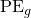and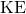, we obtain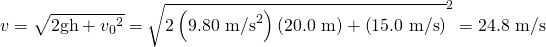If the energy in fusion bombs were used to supply the energy needs of the world, how many of the 9-megaton variety would be needed for a year’s supply of energy (using data from [link])? This is not as far-fetched as it may sound—there are thousands of nuclear bombs, and their energy can be trapped in underground explosions and converted to electricity, as natural geothermal energy is.

(a) Use of hydrogen fusion to supply energy is a dream that may be realized in the next century. Fusion would be a relatively clean and almost limitless supply of energy, as can be seen from [link]. To illustrate this, calculate how many years the present energy needs of the world could be supplied by one millionth of the oceans’ hydrogen fusion energy. (b) How does this time compare with historically significant events, such as the duration of stable economic systems?

(a)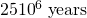(b) This is much, much longer than human time scales.

## Footnotes

1. 1 Representative values

## Glossary

law of conservation of energy
the general law that total energy is constant in any process; energy may change in form or be transferred from one system to another, but the total remains the same
electrical energy
the energy carried by a flow of charge
chemical energy
the energy in a substance stored in the bonds between atoms and molecules that can be released in a chemical reaction